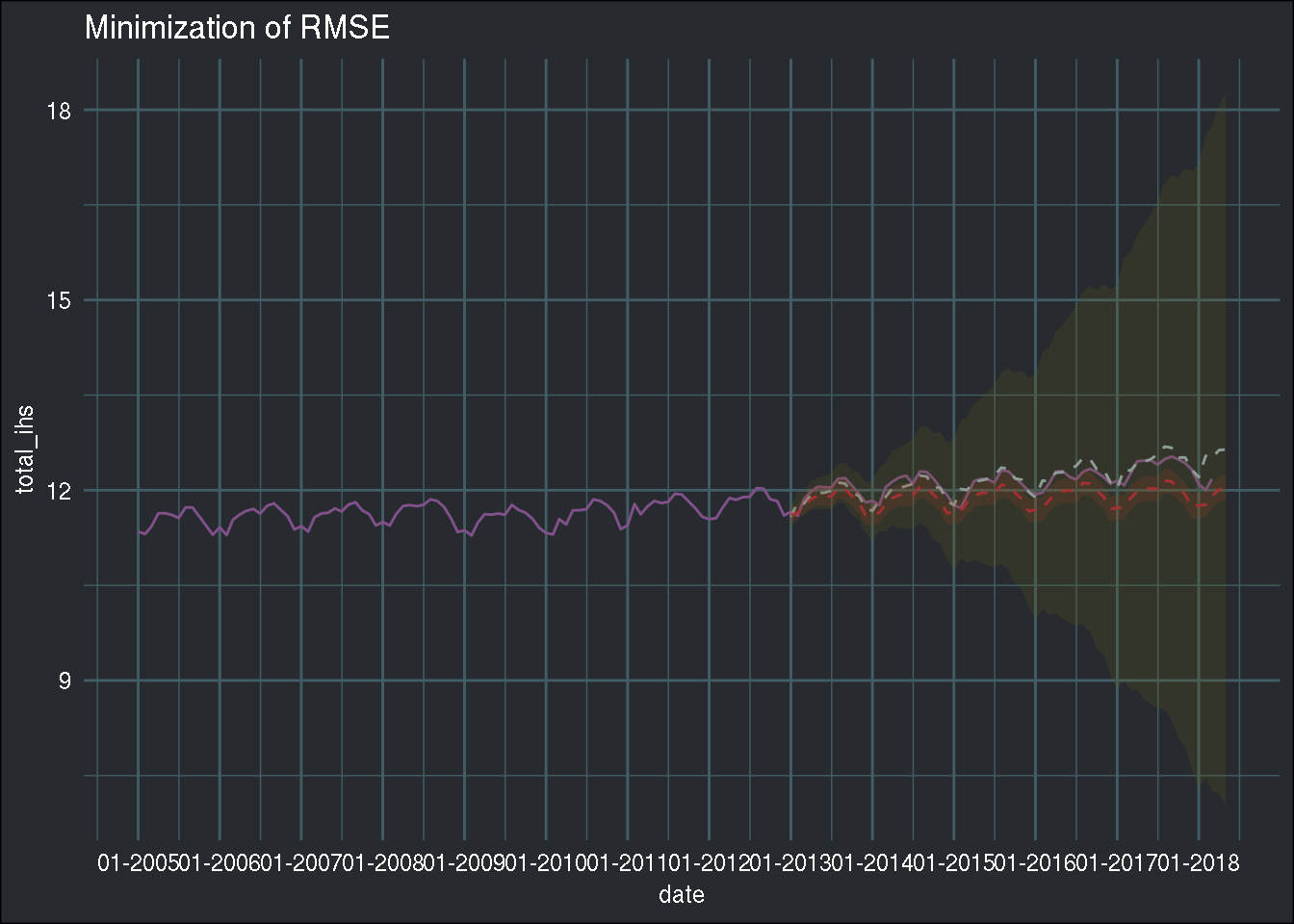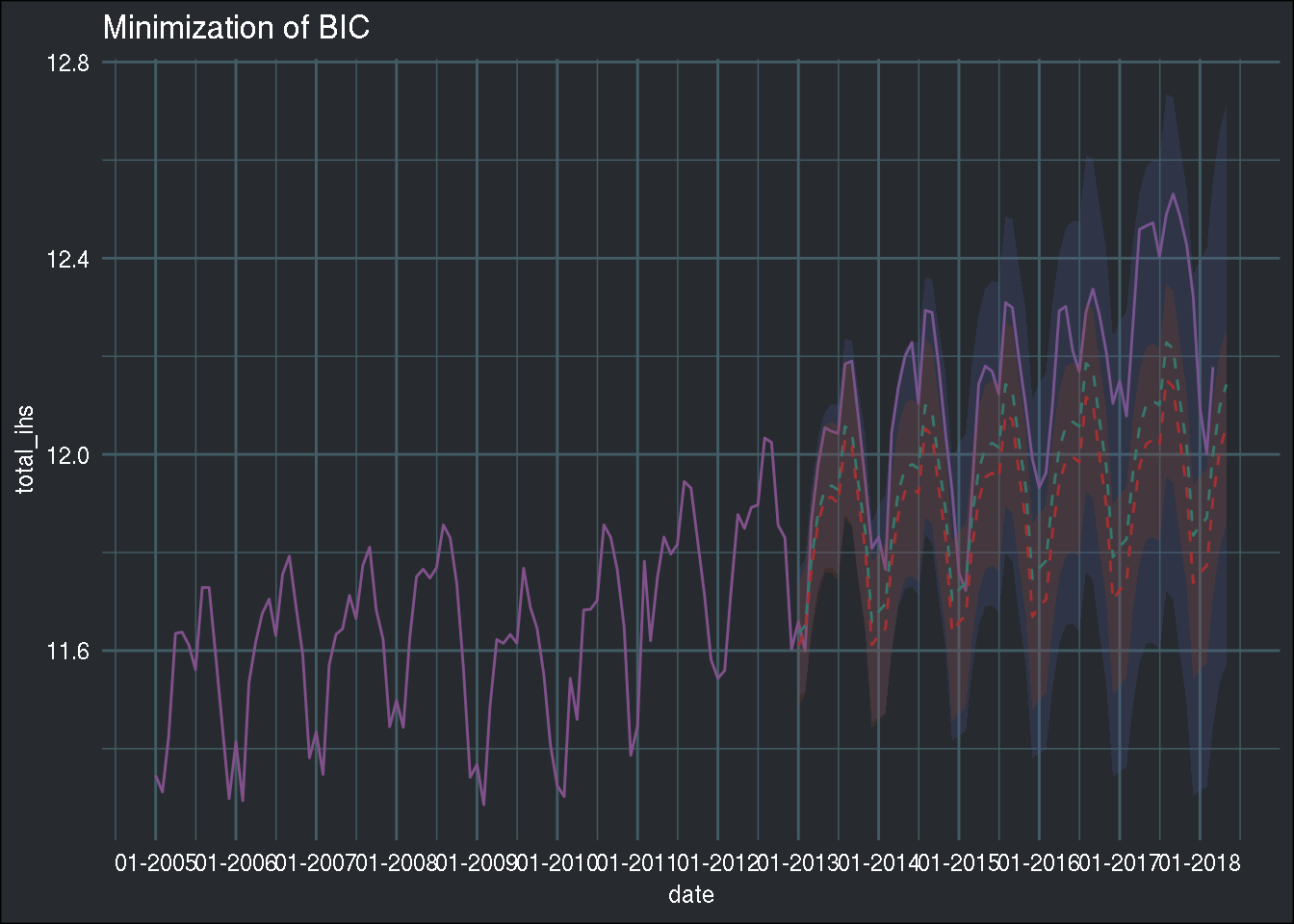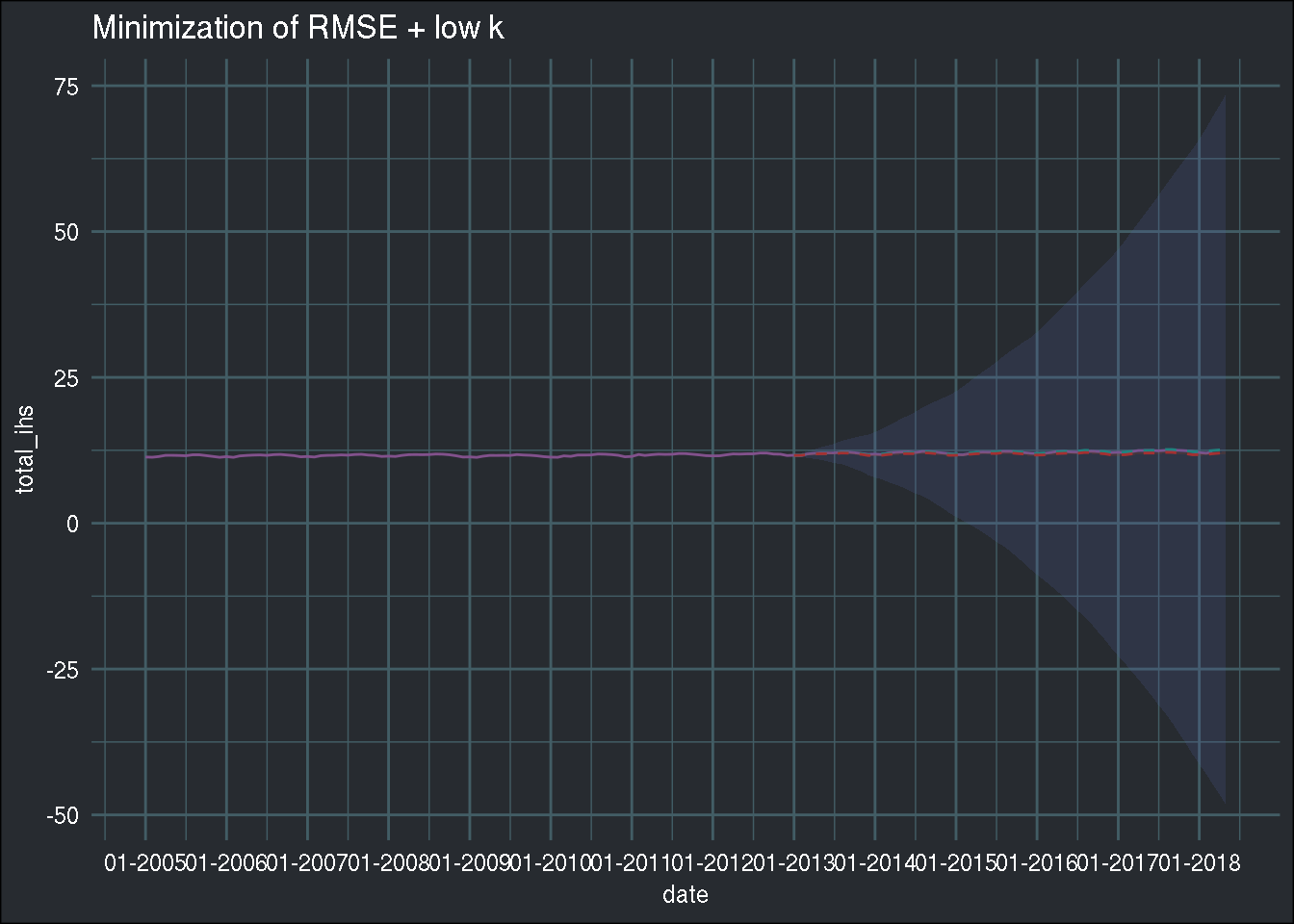Have a question about one of the blog posts? Chat here with me.

# Using a genetic algorithm for the hyperparameter optimization of a SARIMA model

R

## Introduction

In this blog post, I’ll use the data that I cleaned in a previous blog post, which you can download here. If you want to follow along, download the monthly data. In my last blog post I showed how to perform a grid search the “tidy” way. As an example, I looked for the right hyperparameters of a SARIMA model. However, the goal of the post was not hyperparameter optimization per se, so I did not bother with tuning the hyperparameters on a validation set, and used the test set for both validation of the hyperparameters and testing the forecast. Of course, this is not great because doing this might lead to overfitting the hyperparameters to the test set. So in this blog post I split my data into trainig, validation and testing sets and use a genetic algorithm to look for the hyperparameters. Again, this is not the most optimal way to go about this problem, since the `{forecast}` package contains the very useful `auto.arima()` function. I just wanted to see what kind of solution a genetic algorithm would return, and also try different cost functions. If you’re interested, read on!

## Setup

Let’s first load some libraries and define some helper functions (the helper functions were explained in the previous blog posts):

``````library(tidyverse)
library(forecast)
library(rgenoud)
library(parallel)
library(lubridate)
library(furrr)
library(tsibble)
library(brotools)

ihs <- function(x){
log(x + sqrt(x**2 + 1))
}

to_tibble <- function(forecast_object){
point_estimate <- forecast_object\$mean %>%
as_tsibble() %>%
rename(point_estimate = value,
date = index)

upper <- forecast_object\$upper %>%
as_tsibble() %>%
rename(date = index,
upper80 = `80%`,
upper95 = `95%`)

lower <- forecast_object\$lower %>%
as_tsibble() %>%
rename(date = index,
lower80 = `80%`,
lower95 = `95%`)

reduce(list(point_estimate, upper, lower), full_join)
}``````

``avia_clean_monthly <- read_csv("https://raw.githubusercontent.com/b-rodrigues/avia_par_lu/master/avia_clean_monthy.csv")``
``````## Parsed with column specification:
## cols(
##   destination = col_character(),
##   date = col_date(format = ""),
##   passengers = col_double()
## )``````

Let’s split the data into a train set, a validation set and a test set:

``````avia_clean_train <- avia_clean_monthly %>%
select(date, passengers) %>%
filter(year(date) < 2013) %>%
group_by(date) %>%
summarise(total_passengers = sum(passengers)) %>%
pull(total_passengers) %>%
ts(., frequency = 12, start = c(2005, 1))

avia_clean_validation <- avia_clean_monthly %>%
select(date, passengers) %>%
filter(between(year(date), 2013, 2016)) %>%
group_by(date) %>%
summarise(total_passengers = sum(passengers)) %>%
pull(total_passengers) %>%
ts(., frequency = 12, start = c(2013, 1))

avia_clean_test <- avia_clean_monthly %>%
select(date, passengers) %>%
filter(year(date) >= 2016) %>%
group_by(date) %>%
summarise(total_passengers = sum(passengers)) %>%
pull(total_passengers) %>%
ts(., frequency = 12, start = c(2016, 1))

logged_test_data <- ihs(avia_clean_test)

logged_validation_data <- ihs(avia_clean_validation)

logged_train_data <- ihs(avia_clean_train)``````

I will train the models on data from 2005 to 2012, look for the hyperparameters on data from 2013 to 2016 and test the accuracy on data from 2016 to March 2018. For this kind of exercise, the ideal situation would be to perform cross-validation. Doing this with time-series data is not obvious because of the autocorrelation between observations, which would be broken by sampling independently which is required by CV. Also, if for example you do leave-one-out CV, you would end up trying to predict a point in, say, 2017, with data from 2018, which does not make sense. So you should be careful about that. `{forecast}` is able to perform CV for time series and `scikit-learn`, the Python package, is able to perform cross-validation of time series data too. I will not do it in this blog post and simply focus on the genetic algorithm part.

Let’s start by defining the cost function to minimize. I’ll try several, in the first one I will minimize the RMSE:

``````cost_function_rmse <- function(param, train_data, validation_data, forecast_periods){
order <- param[1:3]
season <- c(param[4:6], 12)
model <- purrr::possibly(arima, otherwise = NULL)(x = train_data, order = order,
seasonal = season,
method = "ML")
if(is.null(model)){
return(9999999)
} else {
forecast_model <- forecast::forecast(model, h = forecast_periods)
point_forecast <- forecast_model\$mean
sqrt(mean(point_forecast - validation_data) ** 2)
}
}``````

If `arima()` is not able to estimate a model for the given parameters, I force it to return `NULL`, and in that case force the cost function to return a very high cost. If a model was successfully estimated, then I compute the RMSE.

Let’s also take a look at what `auto.arima()` says:

``````starting_model <- auto.arima(logged_train_data)
summary(starting_model)``````
``````## Series: logged_train_data
## ARIMA(3,0,0)(0,1,1) with drift
##
## Coefficients:
##          ar1     ar2     ar3     sma1   drift
##       0.2318  0.2292  0.3661  -0.8498  0.0029
## s.e.  0.1016  0.1026  0.1031   0.2101  0.0010
##
## sigma^2 estimated as 0.004009:  log likelihood=107.98
## AIC=-203.97   AICc=-202.88   BIC=-189.38
##
## Training set error measures:
##                        ME       RMSE        MAE         MPE      MAPE
## Training set 0.0009924108 0.05743719 0.03577996 0.006323241 0.3080978
##                   MASE        ACF1
## Training set 0.4078581 -0.02707016``````

Let’s compute the cost at this vector of parameters:

``````cost_function_rmse(c(1, 0, 2, 2, 1, 0),
train_data = logged_train_data,
validation_data = logged_validation_data,
forecast_periods = 65)``````
``##  0.1731473``

Ok, now let’s start with optimizing the hyperparameters. Let’s help the genetic algorithm a little bit by defining where it should perform the search:

``domains <- matrix(c(0, 3, 0, 2, 0, 3, 0, 3, 0, 2, 0, 3), byrow = TRUE, ncol = 2)``

This matrix constraints the first parameter to lie between 0 and 3, the second one between 0 and 2, and so on.

Let’s call the `genoud()` function from the `{rgenoud}` package, and use 8 cores:

``````cl <- makePSOCKcluster(8)
clusterExport(cl, c('logged_train_data', 'logged_validation_data'))

tic <- Sys.time()

auto_arima_rmse <- genoud(cost_function_rmse,
nvars = 6,
data.type.int = TRUE,
starting.values = c(1, 0, 2, 2, 1, 0), # <- from auto.arima
Domains = domains,
cluster = cl,
train_data = logged_train_data,
validation_data = logged_validation_data,
forecast_periods = length(logged_validation_data),
hard.generation.limit = TRUE)
toc_rmse <- Sys.time() - tic``````

`makePSOCKcluster()` is a function from the `{parallel}` package. I must also export the global variables `logged_train_data` or `logged_validation_data`. If I don’t do that, the workers called by `genoud()` will not know about these variables and an error will be returned. The option `data.type.int = TRUE` force the algorithm to look only for integers, and `hard.generation.limit = TRUE` forces the algorithm to stop after 100 generations.

The process took 7 minutes, which is faster than doing the grid search. What was the solution found?

``auto_arima_rmse``
``````## \$value
##  0.0001863039
##
## \$par
##  3 2 1 1 2 1
##
##  NA NA NA NA NA NA
##
## \$generations
##  11
##
## \$peakgeneration
##  1
##
## \$popsize
##  1000
##
## \$operators
##  122 125 125 125 125 126 125 126   0``````

Let’s train the model using the `arima()` function at these parameters:

``````best_model_rmse <- arima(logged_train_data, order = auto_arima_rmse\$par[1:3],
season = list(order = auto_arima_rmse\$par[4:6], period = 12),
method = "ML")

summary(best_model_rmse)``````
``````##
## Call:
## arima(x = logged_train_data, order = auto_arima_rmse\$par[1:3], seasonal = list(order = auto_arima_rmse\$par[4:6],
##     period = 12), method = "ML")
##
## Coefficients:
##           ar1      ar2      ar3      ma1     sar1     sma1
##       -0.6999  -0.4541  -0.0476  -0.9454  -0.4996  -0.9846
## s.e.   0.1421   0.1612   0.1405   0.1554   0.1140   0.2193
##
## sigma^2 estimated as 0.006247:  log likelihood = 57.34,  aic = -100.67
##
## Training set error measures:
##                         ME       RMSE        MAE          MPE      MAPE
## Training set -0.0006142355 0.06759545 0.04198561 -0.005408262 0.3600483
##                   MASE         ACF1
## Training set 0.4386693 -0.008298546``````

Let’s extract the forecasts:

``````best_model_rmse_forecast <- forecast::forecast(best_model_rmse, h = 65)

best_model_rmse_forecast <- to_tibble(best_model_rmse_forecast)``````
``````## Joining, by = "date"
## Joining, by = "date"``````
``````starting_model_forecast <- forecast(starting_model, h = 65)

starting_model_forecast <- to_tibble(starting_model_forecast)``````
``````## Joining, by = "date"
## Joining, by = "date"``````

and plot the forecast to see how it looks:

``````avia_clean_monthly %>%
group_by(date) %>%
summarise(total = sum(passengers)) %>%
mutate(total_ihs = ihs(total)) %>%
ggplot() +
ggtitle("Minimization of RMSE") +
geom_line(aes(y = total_ihs, x = date), colour = "#82518c") +
scale_x_date(date_breaks = "1 year", date_labels = "%m-%Y") +
geom_ribbon(data = best_model_rmse_forecast, aes(x = date, ymin = lower95, ymax = upper95),
fill = "#666018", alpha = 0.2) +
geom_line(data = best_model_rmse_forecast, aes(x = date, y = point_estimate),
linetype = 2, colour = "#8e9d98") +
geom_ribbon(data = starting_model_forecast, aes(x = date, ymin = lower95, ymax = upper95),
fill = "#98431e", alpha = 0.2) +
geom_line(data = starting_model_forecast, aes(x = date, y = point_estimate),
linetype = 2, colour = "#a53031") +
theme_blog()``````The yellowish line and confidence intervals come from minimizing the genetic algorithm, and the redish from `auto.arima()`. Interesting; the point estimate is very precise, but the confidence intervals are very wide. Low bias, high variance.

Now, let’s try with another cost function, where I minimize the BIC, similar to the `auto.arima()` function:

``````cost_function_bic <- function(param, train_data, validation_data, forecast_periods){
order <- param[1:3]
season <- c(param[4:6], 12)
model <- purrr::possibly(arima, otherwise = NULL)(x = train_data, order = order,
seasonal = season,
method = "ML")
if(is.null(model)){
return(9999999)
} else {
BIC(model)
}
}``````

Let’s take a look at the cost at the parameter values returned by `auto.arima()`:

``````cost_function_bic(c(1, 0, 2, 2, 1, 0),
train_data = logged_train_data,
validation_data = logged_validation_data,
forecast_periods = 65)``````
``##  -184.6397``

Let the genetic algorithm run again:

``````cl <- makePSOCKcluster(8)
clusterExport(cl, c('logged_train_data', 'logged_validation_data'))

tic <- Sys.time()

auto_arima_bic <- genoud(cost_function_bic,
nvars = 6,
data.type.int = TRUE,
starting.values = c(1, 0, 2, 2, 1, 0), # <- from auto.arima
Domains = domains,
cluster = cl,
train_data = logged_train_data,
validation_data = logged_validation_data,
forecast_periods = length(logged_validation_data),
hard.generation.limit = TRUE)
toc_bic <- Sys.time() - tic``````

This time, it took 6 minutes, a bit slower than before. Let’s take a look at the solution:

``auto_arima_bic``
``````## \$value
##  -201.0656
##
## \$par
##  0 1 1 1 0 1
##
##  NA NA NA NA NA NA
##
## \$generations
##  12
##
## \$peakgeneration
##  1
##
## \$popsize
##  1000
##
## \$operators
##  122 125 125 125 125 126 125 126   0``````

Let’s train the model at these parameters:

``````best_model_bic <- arima(logged_train_data, order = auto_arima_bic\$par[1:3],
season = list(order = auto_arima_bic\$par[4:6], period = 12),
method = "ML")

summary(best_model_bic)``````
``````##
## Call:
## arima(x = logged_train_data, order = auto_arima_bic\$par[1:3], seasonal = list(order = auto_arima_bic\$par[4:6],
##     period = 12), method = "ML")
##
## Coefficients:
##           ma1    sar1    sma1
##       -0.6225  0.9968  -0.832
## s.e.   0.0835  0.0075   0.187
##
## sigma^2 estimated as 0.004145:  log likelihood = 109.64,  aic = -211.28
##
## Training set error measures:
##                       ME       RMSE        MAE        MPE      MAPE
## Training set 0.003710982 0.06405303 0.04358164 0.02873561 0.3753513
##                   MASE        ACF1
## Training set 0.4553447 -0.03450603``````

And let’s plot the results:

``````best_model_bic_forecast <- forecast::forecast(best_model_bic, h = 65)

best_model_bic_forecast <- to_tibble(best_model_bic_forecast)``````
``````## Joining, by = "date"
## Joining, by = "date"``````
``````avia_clean_monthly %>%
group_by(date) %>%
summarise(total = sum(passengers)) %>%
mutate(total_ihs = ihs(total)) %>%
ggplot() +
ggtitle("Minimization of BIC") +
geom_line(aes(y = total_ihs, x = date), colour = "#82518c") +
scale_x_date(date_breaks = "1 year", date_labels = "%m-%Y") +
geom_ribbon(data = best_model_bic_forecast, aes(x = date, ymin = lower95, ymax = upper95),
fill = "#5160a0", alpha = 0.2) +
geom_line(data = best_model_bic_forecast, aes(x = date, y = point_estimate),
linetype = 2, colour = "#208480") +
geom_ribbon(data = starting_model_forecast, aes(x = date, ymin = lower95, ymax = upper95),
fill = "#98431e", alpha = 0.2) +
geom_line(data = starting_model_forecast, aes(x = date, y = point_estimate),
linetype = 2, colour = "#a53031") +
theme_blog()``````The solutions are very close, both in terms of point estimates and confidence intervals. Bias increased, but variance lowered… This gives me an idea! What if I minimize the RMSE, while keeping the number of parameters low, as a kind of regularization? This is somewhat what minimising BIC does, but let’s try to do it a more “naive” approach:

``````cost_function_rmse_low_k <- function(param, train_data, validation_data, forecast_periods, max.order){
order <- param[1:3]
season <- c(param[4:6], 12)
if(param + param + param + param > max.order){
return(9999999)
} else {
model <- purrr::possibly(arima, otherwise = NULL)(x = train_data,
order = order,
seasonal = season,
method = "ML")
}
if(is.null(model)){
return(9999999)
} else {
forecast_model <- forecast::forecast(model, h = forecast_periods)
point_forecast <- forecast_model\$mean
sqrt(mean(point_forecast - validation_data) ** 2)
}
}``````

This is also similar to what `auto.arima()` does; by default, the `max.order` argument in `auto.arima()` is set to 5, and is the sum of `p + q + P + Q`. So I’ll try something similar.

Let’s take a look at the cost at the parameter values returned by `auto.arima()`:

``````cost_function_rmse_low_k(c(1, 0, 2, 2, 1, 0),
train_data = logged_train_data,
validation_data = logged_validation_data,
forecast_periods = 65,
max.order = 5)``````
``##  0.1731473``

Let’s see what will happen:

``````cl <- makePSOCKcluster(8)
clusterExport(cl, c('logged_train_data', 'logged_validation_data'))

tic <- Sys.time()

auto_arima_rmse_low_k <- genoud(cost_function_rmse_low_k,
nvars = 6,
data.type.int = TRUE,
starting.values = c(1, 0, 2, 2, 1, 0), # <- from auto.arima
max.order = 5,
Domains = domains,
cluster = cl,
train_data = logged_train_data,
validation_data = logged_validation_data,
forecast_periods = length(logged_validation_data),
hard.generation.limit = TRUE)
toc_rmse_low_k <- Sys.time() - tic``````

It took 1 minute to train this one, quite fast! Let’s take a look:

``auto_arima_rmse_low_k``
``````## \$value
##  0.002503478
##
## \$par
##  1 2 0 3 1 0
##
##  NA NA NA NA NA NA
##
## \$generations
##  11
##
## \$peakgeneration
##  1
##
## \$popsize
##  1000
##
## \$operators
##  122 125 125 125 125 126 125 126   0``````

And let’s plot it:

``````best_model_rmse_low_k <- arima(logged_train_data, order = auto_arima_rmse_low_k\$par[1:3],
season = list(order = auto_arima_rmse_low_k\$par[4:6], period = 12),
method = "ML")

summary(best_model_rmse_low_k)``````
``````##
## Call:
## arima(x = logged_train_data, order = auto_arima_rmse_low_k\$par[1:3], seasonal = list(order = auto_arima_rmse_low_k\$par[4:6],
##     period = 12), method = "ML")
##
## Coefficients:
##           ar1     sar1     sar2     sar3
##       -0.6468  -0.7478  -0.5263  -0.1143
## s.e.   0.0846   0.1171   0.1473   0.1446
##
## sigma^2 estimated as 0.01186:  log likelihood = 57.88,  aic = -105.76
##
## Training set error measures:
##                        ME      RMSE        MAE         MPE      MAPE
## Training set 0.0005953302 0.1006917 0.06165919 0.003720452 0.5291736
##                   MASE       ACF1
## Training set 0.6442205 -0.3706693``````
``````best_model_rmse_low_k_forecast <- forecast::forecast(best_model_rmse_low_k, h = 65)

best_model_rmse_low_k_forecast <- to_tibble(best_model_rmse_low_k_forecast)``````
``````## Joining, by = "date"
## Joining, by = "date"``````
``````avia_clean_monthly %>%
group_by(date) %>%
summarise(total = sum(passengers)) %>%
mutate(total_ihs = ihs(total)) %>%
ggplot() +
ggtitle("Minimization of RMSE + low k") +
geom_line(aes(y = total_ihs, x = date), colour = "#82518c") +
scale_x_date(date_breaks = "1 year", date_labels = "%m-%Y") +
geom_ribbon(data = best_model_rmse_low_k_forecast, aes(x = date, ymin = lower95, ymax = upper95),
fill = "#5160a0", alpha = 0.2) +
geom_line(data = best_model_rmse_low_k_forecast, aes(x = date, y = point_estimate),
linetype = 2, colour = "#208480") +
geom_ribbon(data = starting_model_forecast, aes(x = date, ymin = lower95, ymax = upper95),
fill = "#98431e", alpha = 0.2) +
geom_line(data = starting_model_forecast, aes(x = date, y = point_estimate),
linetype = 2, colour = "#a53031") +
theme_blog()``````Looks like this was not the right strategy. There might be a better cost function than what I have tried, but looks like minimizing the BIC is the way to go.

Hope you enjoyed! If you found this blog post useful, you might want to follow me on twitter for blog post updates or buy me an espresso.